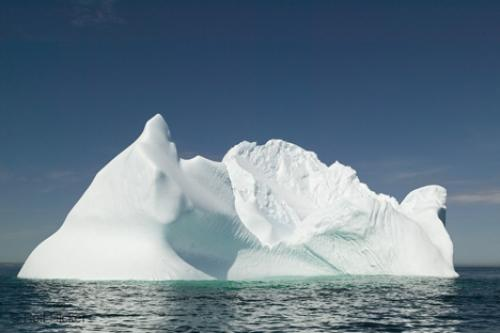# Iceberg floating in the oceanAn iceberg having a specific gravity of $0.92$ is floating on the salt water of specific gravity of $1.03$. If the volume of the iceberg above the water surface is $1000~m^3$, which of the following is the most approximate total volume of the iceberg in $m^3$?

Details:

unit weight of water = $9.81\frac{kN}{m^3}$

×

Problem Loading...

Note Loading...

Set Loading...